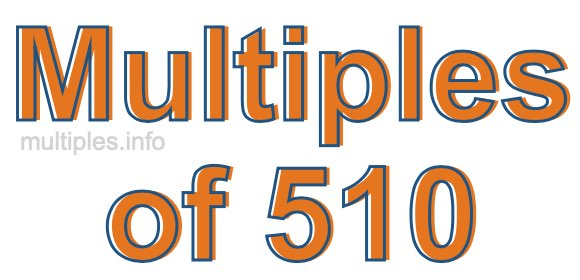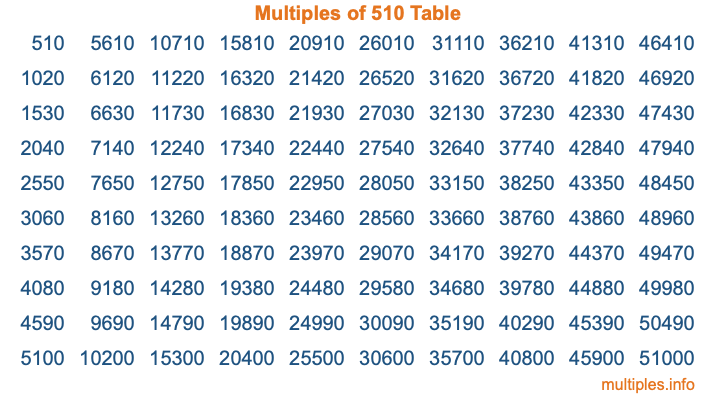Multiples of 510Welcome to the Multiples of 510 page. Here we will first teach you everything you will ever need to know about the multiples of 510, and then give you a study guide summary of everything we taught you to make sure you remember it all. Use this page to look up facts and learn information about the multiples of 510. This page will make you a multiples of five hundred ten expert!

Definition of Multiples of 510
Multiples of 510 are all the numbers that when divided by 510 equal an integer. Each of the multiples of 510 are called a multiple. A multiple of 510 is created by multiplying 510 by an integer.

Therefore, to create a list of multiples of 510, you start with 1 multiplied by 510, then 2 multiplied by 510, then 3 multiplied by 510, and so on for as long as you want. Thus, the list of the first five multiples of 510 is 510, 1020, 1530, 2040, and 2550. To see a larger list of multiples of 510, see the printable image of Multiples of 510 further down on this page. We also have a category where you can choose any nth multiple of 510.

Multiples of 510 Checker
The Multiples of 510 Checker below checks to see if any number of your choice is a multiple of 510. In other words, it checks to see if there is any number (integer) that when multiplied by 510 will equal your number. To do that, we divide your number by 510. If the the quotient is an integer, then your number is a multiple of 510.

Is  a multiple of 510?

Least Common Multiple of 510 and ...
A Least Common Multiple (LCM) is the lowest multiple that two or more numbers have in common. This is also called the smallest common multiple or lowest common multiple and is useful to know when you are adding our subtracting fractions. Enter one or more numbers below (510 is already entered) to find the LCM.

Check out our LCM Calculator if you need more details about the Least Common Multiple or if you need the LCM for different numbers for adding and subtraction fractions.

nth Multiple of 510
As we stated above, 510 is the first multiple of 510, 1020 is the second multiple of 510, 1530 is the third multiple of 510, and so on. Enter a number below to find the nth multiple of 510.

th multiple of 510

Multiples of 510 vs Factors of 510
510 is a multiple of 510 and a factor of 510, but that is where the similarities end. All postive multiples of 510 are 510 or greater than 510. All positive factors of 510 are 510 or less than 510.

Below is the beginning list of multiples of 510 and the factors of 510 so you can compare:

Multiples of 510: 510, 1020, 1530, 2040, 2550, etc.

Factors of 510: 1, 2, 3, 5, 6, 10, 15, 17, 30, 34, 51, 85, 102, 170, 255, 510

As you can see, the multiples of 510 are all the numbers that you can divide by 510 to get a whole number. The factors of 510, on the other hand, are all the whole numbers that you can multiply by another whole number to get 510.

It's also interesting to note that if a number (x) is a factor of 510, then 510 will also be a multiple of that number (x).

Multiples of 510 vs Divisors of 510
The divisors of 510 are all the integers that 510 can be divided by evenly. Below is a list of the divisors of 510.

Divisors of 510: 1, 2, 3, 5, 6, 10, 15, 17, 30, 34, 51, 85, 102, 170, 255, 510

The interesting thing to note here is that if you take any multiple of 510 and divide it by a divisor of 510, you will see that the quotient is an integer.

Multiples of 510 Table
Below is an image of the first 100 multiples of 510 in a table. The table is in chronological order, column by column. The first column has the first ten multiples of 510, the second column has the next ten multiples of 510, and so on.The Multiples of 510 Table is also referred to as the 510 Times Table or Times Table of 510. You are welcome to print out our table for your studies.

Negative Multiples of 510
Although not often discussed or needed in math, it is worth mentioning that you can make a list of negative multiples of 510 by multiplying 510 by -1, then by -2, then by -3, and so on, to get the following list of negative multiples of 510:

-510, -1020, -1530, -2040, -2550, etc.

Multiples of 510 Summary
Below is a summary of important Multiples of 510 facts that we have discussed on this page. To retain the knowledge on this page, we recommend that you read through the summary and explain to yourself or a study partner why they hold true.

There are an infinite number of multiples of 510.

A multiple of 510 divided by 510 will equal a whole number.

510 divided by a factor of 510 equals a divisor of 510.

The nth multiple of 510 is n times 510.

The largest factor of 510 is equal to the first positive multiple of 510.

510 is a multiple of every factor of 510.

510 is a multiple of 510.

A multiple of 510 divided by a divisor of 510 equals an integer.

510 divided by a divisor of 510 equals a factor of 510.

Any integer times 510 will equal a multiple of 510.

Multiples of a Number
Here you can get the multiples of another number, all with the same attention to detail as we did for multiples of 510 on this page.

Multiples of
Multiples of 511
Did you find our page about multiples of five hundred ten educational? Do you want more knowledge? Check out the multiples of the next number on our list!